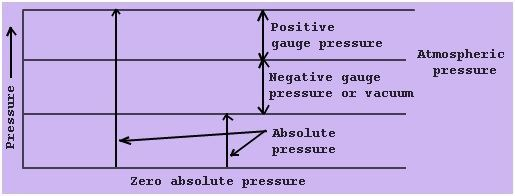# Absolute Pressure Formula

## What is Absolute Pressure?

When any pressure is detected above the absolute zero of pressure, it is labelled as absolute pressure. It is measured using a barometer, and it is equal to measuring pressure plus the atmospheric pressure.Diagram showing absolute pressure, vacuum and gauge

Absolute pressure formula (pabs) is given by,

Pabs=Patm+Pgauge

where pgauge is the gauge pressure

patm is atmospheric pressure.

The vacuum pressure is articulated as,

Vacuum Pressure = Atmospheric Pressure – Absolute Pressure

At sea level, it is around 14.7 pounds per square inch.

## Solved Examples

Let’s see some examples of absolute pressure:

Problem 1: A pressure gauge measures the pgauge reading as 31 psi. If the atmospheric pressure is 14.2 psi. Compute the absolute pressure that corresponds to pgauge reading.

Given:

patm (Atmospheric pressure) = 14.2 psi
pgauge (Gauge pressure) = 31 psi
Absolute pressure (pabs) =  patm + pgauge
= 14.2 psi + 31 psi
pgauge= 45.2 psi

Problem 2: The psia pressure instrument gives the reading as 35.8 psi. If the atmospheric pressure is 15 psi, calculate the corresponding guage pressure.